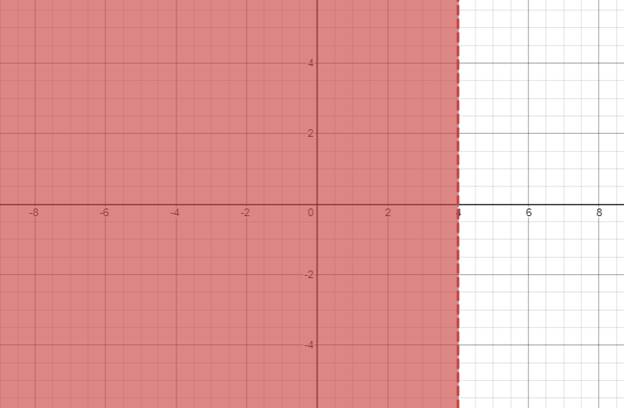# The solution of given nonlinear inequality equation and plot a graph for it.### Precalculus: Mathematics for Calcu...

6th Edition
Stewart + 5 others
Publisher: Cengage Learning
ISBN: 9780840068071### Precalculus: Mathematics for Calcu...

6th Edition
Stewart + 5 others
Publisher: Cengage Learning
ISBN: 9780840068071

#### Solutions

Chapter 1.7, Problem 51E
To determine

## The solution of given nonlinear inequality equation and plot a graph for it.

Expert Solution

x<4,x>2 and x>2

### Explanation of Solution

Given:

Non-linear inequality equation

(x4)(x+2)2<0

Calculation,

Non-linear inequality equation,

(x4)(x+2)2<0(x4)(x+2)(x+2)<0Now,x4<0x<4andx+2<0x>2andx+2<0x>2

Now, graph of (x4)(x+2)2<0Conclusion:

Hence, the values of x is x<4,x>2 and x>2 .

### Have a homework question?

Subscribe to bartleby learn! Ask subject matter experts 30 homework questions each month. Plus, you’ll have access to millions of step-by-step textbook answers!